# Decimal - Definition with Examples

The Complete K-5 Math Learning Program Built for Your Child

• 40 Million Kids

Loved by kids and parent worldwide

• 50,000 Schools

Trusted by teachers across schools

• Comprehensive Curriculum

Aligned to Common Core

## Decimal Games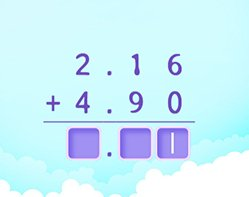Work with visual models to gain understanding of decimal addition. Extend knowledge of multi digit addition to adding decimals (up to hundredths place)

Covers Common Core Curriculum 5.NBT.7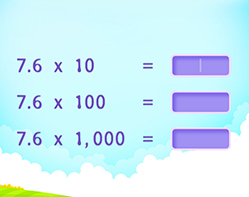Multiply Decimals by a Multiple of 10

Identify the pattern in the placement of the decimal point, when a decimal is multiplied by a power of 10. At times you may need to append zeroes in the product.

Covers Common Core Curriculum 5.NBT.7

## What is a Decimal?

In algebra, a decimal number can be defined as a number whose whole number part and the fractional part is separated by a decimal point. The dot in a decimal number is called a decimal point. The digits following the decimal point show a value smaller than one.

Here's an example of a decimal number 17.48, in which 17 is the whole number, while 48 is the decimal part.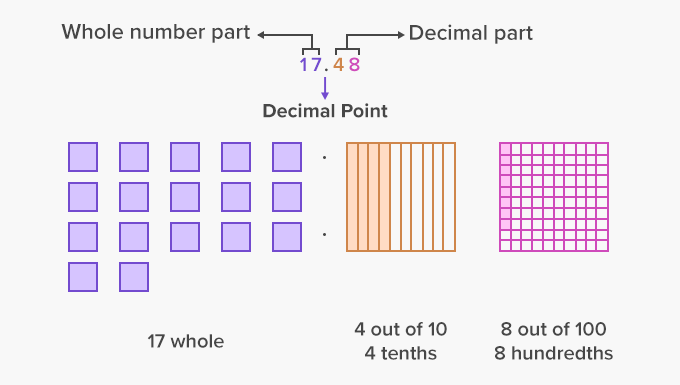Decimals are based on the preceding powers of 10. Thus, as we move from left to right, the place value of digits gets divided by 10, meaning the decimal place value determines the tenths, hundredths and thousandths. A tenth means one tenth or 1/10. In decimal form, it is 0.1. Hundredth means 1/100. In decimal form, it is 0.01.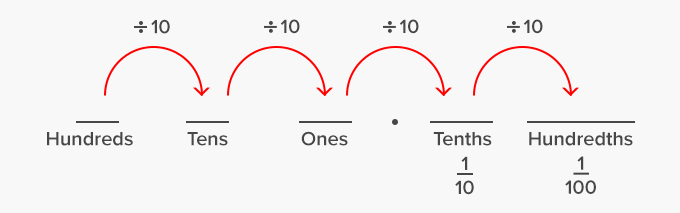Here’s an example of how the fractional part can be converted into decimals.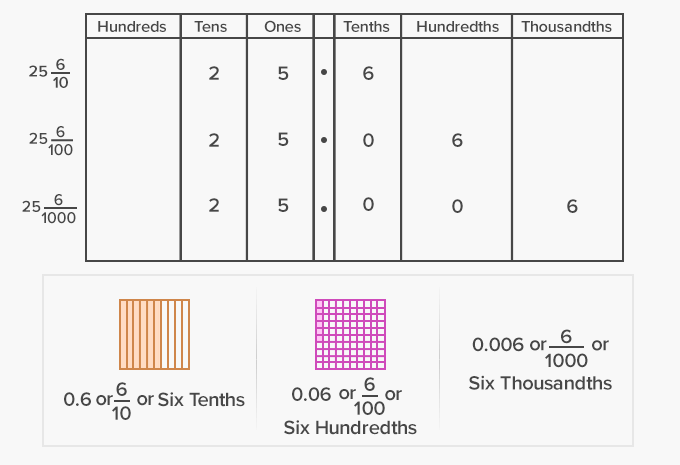Decimals can be written both in expanded form and in words.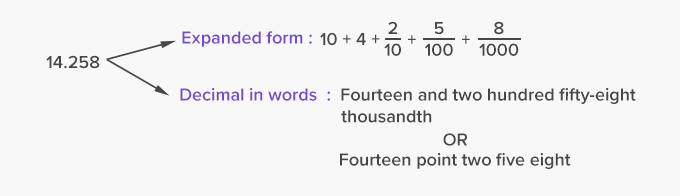Tenths, hundredths, and thousandths can be represented on a number line. To represent tenths, the distance between each whole number on a number line is partitioned into 10 equal parts where each part represents a tenth.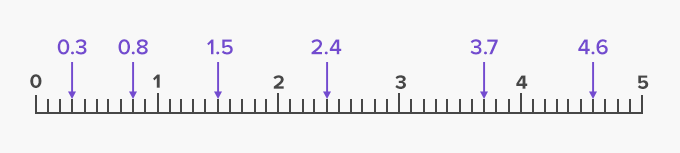Fun Facts Decimal fractions were first developed and used by the Chinese in the end of 4th century BC, and then spread to the Middle East and from there to Europe.

##Let's do it!

Instead of teaching decimals and then handing out practice worksheets to your children, give them real life instances in which they can use decimals or convert numbers to decimals. For instance, while shopping, ask your child to compute the price for an item in decimals (half a dozen apples).

##Related math vocabulary

Won Numerous Awards & Honors